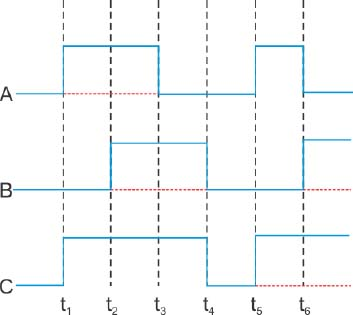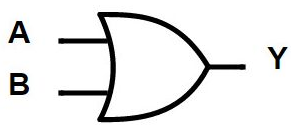#### The figure shows a logic circuit with two inputs A and B and the output C. The voltage wave forms across A, B and C are as given. The logic circuit gate is:Option 1) OR gate Option 2) NOR gate Option 3) AND gate Option 4) NAND gateAs we learnt in

OR gate -

Relation between input and output- wherein

A and B are input

Y is out put

From given wavefrom, the following truth table can be made.

 Input Output A                        B    0                        0 1                        0 1                        1 0                        1 C 0 1 1 1

This truth table obtained is of OR gate . Hence logic gate is OR gate.

Option 1)

OR gate

This is correct option

Option 2)

NOR gate

This is incorrect option

Option 3)

AND gate

This is incorrect option

Option 4)

NAND gate

This is incorrect option